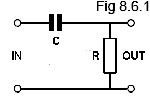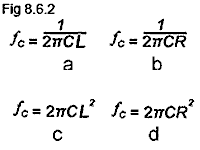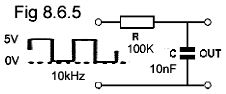# Filter Quiz

Try our quiz, based on the information you can find in Module 8. Submit your answers and see how many you get right, but don't be disappointed if you get answers wrong. Just follow the hints to find the right answer and learn about Filters and Wave Shaping Circuits as you go.

### 1.Refer to Fig 8.6.1. What is this circuit called when used with sinusoidal signals?

### 2.With reference to Fig 8.6.2, which of the formulae would be used to find the corner frequency of a low pass filter?

### 3.

Which of the following labels would most appropriately describe a High pass filter when used in an audio amplifier

### 4.With reference to Fig 8.6.3 what would be the approximate amplitude of the signal at the output?

### 5.Which of the following describes the circuit in Fig 8.6.4?

### 6.What will be the waveform at the output of Fig 8.6.5?

### 7.

A square wave with a periodic time of 10µs is applied to the input of a differentiator circuit. For differentiated pulses to appear at the output, the time constant of the CR network should be approximately:

### 8.

Which of the following networks can be used as a differentiator

### 9.

With reference to Fig 8.6.5, if a DC voltmeter is connected across the output terminals of the circuit with the input shown, what will be the voltmeter reading?

### 10.With reference to Fig 8.6.6, if a triangular wave having a periodic time shorter that the CR time constant of the circuit is applied to the input, the shape of the waveform at the output would be approximately...?
Top of Page.>